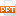# Bài giảng Electromagnetic Fields - Chapter 7 - Trần Quang Việt

P7.2:  The dimension a of an air-dielectric parallel-plate waveguide is 2 cm. A 20 GHz signal is launched in the waveguide: (a) Find all the modes that can propagate at this frequency and their cutoff frequencies ? (b) Find the phase velocities of two TM modes with the lowest cutoff frequencies ? (c) What should be the maximum plate seperation to insure that only one mode propagates at this frequency?

P7.3:  The dimension a of an air-dielectric parallel-plate waveguide is 3 cm. Find the values of q for each of the following cases: (a) f = 6000 MHz, TE10 mode; (b) f = 15000 MHz, TE10 mode ; (c) f = 15000 MHz, TE20 mode.9 trang thamphan 28/12/2022 260
Bạn đang xem tài liệu "Bài giảng Electromagnetic Fields - Chapter 7 - Trần Quang Việt", để tải tài liệu gốc về máy hãy click vào nút Download ở trên.

File đính kèm:

•bai_giang_electromagnetic_fields_chapter_7_tran_quang_viet.ppt

### Nội dung text: Bài giảng Electromagnetic Fields - Chapter 7 - Trần Quang Việt

1. Electromagnetic Fields – Prob – ch_7 P7.1: (Ans: a) 15 GHz b) 2.27 cm ) P7.2: The dimension a of an air-dielectric parallel-plate waveguide is 2 cm. A 20 GHz signal is launched in the waveguide: (a) Find all the modes that can propagate at this frequency and their cutoff frequencies ? (b) Find the phase velocities of two TM modes with the lowest cutoff frequencies ? (c) What should be the maximum plate seperation to insure that only one mode propagates at this frequency? 8 (Ans: (a) TM00(0), TE/TM10(7.5G), TE/TM20(15G) (b) 3&3.23166*10 m/s (c) 0.75cm ) © Trần Quang Việt – BMCS – Khoa Điện – ĐHBK Tp.HCM
2. Electromagnetic Fields – Prob – ch_7 P7.5: Find the spacing a for a parallel-plate waveguide having a dielectric of  = 90 and  = 0 such that 6000 MHz is 20% above the cutoff frequency of the dominant mode , that is , the mode with the lowest cutoff frequency. (Ans: 1 cm ) P7.6: The dimension a of a parallel-plate waveguide with a dielectric of  = 40 and  = 0 is 3 cm. Determine the propagating modes for a wave of frequency 6000 MHz ? For each propagating mode, find c, fc, , and vpz ? © Trần Quang Việt – BMCS – Khoa Điện – ĐHBK Tp.HCM
3. Electromagnetic Fields – Prob – ch_7 P7.9: (Ans: axb = 2.25cm x 1.3cm ) P7.10: Consider propagation of TM waves of frequency f = 6000 MHz in an air-dielectric waveguide of square cross section (b = a) . Find the range of a for which the TM11 mode propagates with a 20% safety factor ( f > 1.2fc) but also such that f is at least 20% below the fc of the next higher-order mode . (Ans: 4.24 cm < a < 4.47 cm ) © Trần Quang Việt – BMCS – Khoa Điện – ĐHBK Tp.HCM
4. Electromagnetic Fields – Prob – ch_7 P7.12: A rectangular waveguide of dimensions a = 4 cm and b = 2 cm has a dielectric discontinuity as shown in Fig. 7.12. A TM11 wave of frequency 10000 Mhz is incident on the discontinuity from the free space side. (a) Find the SWR in the free space section (b) Find the length and the permittivity of a quarter-wave section require to archieve a match between he two media. Assume  = 0 for the quarter-wave section. Section 1 Section 2 (0, 0) (0, 90) Fig. 7.11 z (Ans: (a) 1.7 (b) 0.366 cm, 4.90 ) © Trần Quang Việt – BMCS – Khoa Điện – ĐHBK Tp.HCM
5. Electromagnetic Fields – Prob – ch_7 P7.15: (Ans: a) 0.835 GHz b) 0.515 Np/m c) 0.67m ) © Trần Quang Việt – BMCS – Khoa Điện – ĐHBK Tp.HCM